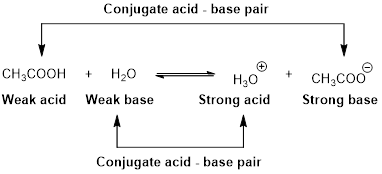Chapter 16, Problem 1PS

Chapter
Section
Textbook Problem

Write the formula and the give the name of the conjugate base of each of the following acids. a) HCN b) HSO 4 − c) HF

(a)

Interpretation Introduction

Interpretation:

Formula and the conjugate base of given acid has to be written.

Concept Introduction:

Arrhenius Acids and Bases

Acid release hydrogen ion in water

Base release hydroxide ions in water.

HCl(aq) H+(aq) + Cl-(aq).........AcidNaOH(aq) Na+(aq)+ OH-(aq)...........Base

An acid is a substance that produces hydronium ions, H3O+ when dissolved in water.

Bronsted –Lowry definitions:

A Bronsted –Lowry acid is a proton donor, it donates a hydrogen ion, H+

A Bronsted-Lowry base is a proton acceptor, it accepts a hydrogen ion H+.Lewis definition:

A Lewis acid is a substance that can accept and share an electron pair.

A Lewis base is a substance that can donate and share an electron pair.Bronsted –Lowry conjugate acid-Base pairs:

When an acid is dissolved in water, the acid (HA) donates a proton to water to form a new acid (conjugate acid) and a new base (conjugate base).The conjugate base of HCN is Cyanide ion (CN-)

Explanation

HCN is an acid .Cyanide ion (CN-) is a conjugate base. HCN and (CN-) is differs by only one proton. Therefore, (CN-) is a conjugate base of HCN

(b)

Interpretation Introduction

Interpretation:

Formula and the conjugate base of given acid has to be written.

Concept Introduction:

Arrhenius Acids and Bases

Acid release hydrogen ion in water

Base release hydroxide ions in water.

HCl(aq) H+(aq) + Cl-(aq).........AcidNaOH(aq) Na+(aq)+ OH-(aq)...........Base

An acid is a substance that produces hydronium ions, H3O+ when dissolved in water.

Bronsted –Lowry definitions:

A Bronsted –Lowry acid is a proton donor, it donates a hydrogen ion, H+

A Bronsted-Lowry base is a proton acceptor, it accepts a hydrogen ion H+.Lewis definition:

A Lewis acid is a substance that can accept and share an electron pair.

A Lewis base is a substance that can donate and share an electron pair.Bronsted –Lowry conjugate acid-Base pairs:

When an acid is dissolved in water, the acid (HA) donates a proton to water to form a new acid (conjugate acid) and a new base (conjugate base).The conjugate base of HSO4- is sulphate ion SO42-.

Explanation

HSO4- is an acid .sulphate ion (SO42-) is a conjugate base. HSO4- and (SO42-) is differs by only one proton. Therefore,( SO42-) ion is a conjugate base of HSO4-

(c)

Interpretation Introduction

Interpretation:

Formula and the conjugate base of given acid has to be written.

Concept Introduction:

Arrhenius Acids and Bases

Acid release hydrogen ion in water

Base release hydroxide ions in water.

HCl(aq) H+(aq) + Cl-(aq).........AcidNaOH(aq) Na+(aq)+ OH-(aq)...........Base

An acid is a substance that produces hydronium ions, H3O+ when dissolved in water.

Bronsted –Lowry definitions:

A Bronsted –Lowry acid is a proton donor, it donates a hydrogen ion, H+

A Bronsted-Lowry base is a proton acceptor, it accepts a hydrogen ion H+.Lewis definition:

A Lewis acid is a substance that can accept and share an electron pair.

A Lewis base is a substance that can donate and share an electron pair.Bronsted –Lowry conjugate acid-Base pairs:

When an acid is dissolved in water, the acid (HA) donates a proton to water to form a new acid (conjugate acid) and a new base (conjugate base).The conjugate base of HF is fluoride ion (F).

Explanation

HF is an acid .Fluoride ion (F-) is a conjugate base. HF and (F-) is differs by only one proton. Therefore, Fluoride (F-) is a conjugate base of HF

The Solution to Your Study Problems

Bartleby provides explanations to thousands of textbook problems written by our experts, many with advanced degrees!

Get Started

The bladder straddles the cardiovascular system and filters the blood. T F

Nutrition: Concepts and Controversies - Standalone book (MindTap Course List)

Explain the structure and function of the endomembrane system.

Biology: The Dynamic Science (MindTap Course List)

Find all the answers to your study problems with bartleby.
Textbook solutions plus Q&A. Get As ASAP arrow_forward